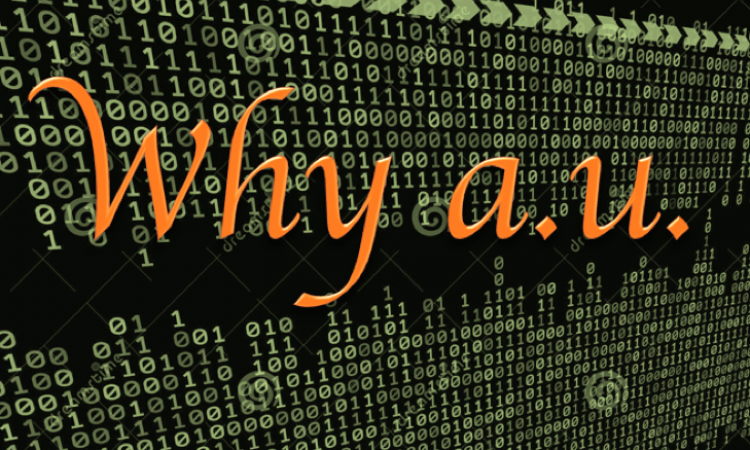# Why atomic units (a.u.) are used in QM calculation?

QM calculation using gaussian, energies are written in atom units(a.u.). However eV, kcal/mol and kJ/mol are used in papers.
Why computational chemistry software are using atom units(a.u.)?

Looking at the log file of the calculation result in Gaussian, all the energy is written in units of a.u. What is the reason not to use eV, kcal / mol, kJ / mol used in articles etc?

#### Using a.u. makes calculations easier!

When a.u.(atomic unit) is used, the following relationship holds.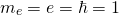That is, the mass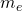of the electron, the charge e, and the converted Planck constant (Dirac constant)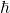all become 1, which greatly facilitates the calculation!

I guess some people might think “For computers, there are no differences between 1 and decimal number.” Actually, computer are no good at calculating decimal numbers.

Computers hold numbers in binary numbers, but most decimals can not be represented exactly in binary numbers. This also affects the accuracy of the calculation.

#### Atomic Units (a.u.) and Conversion Factors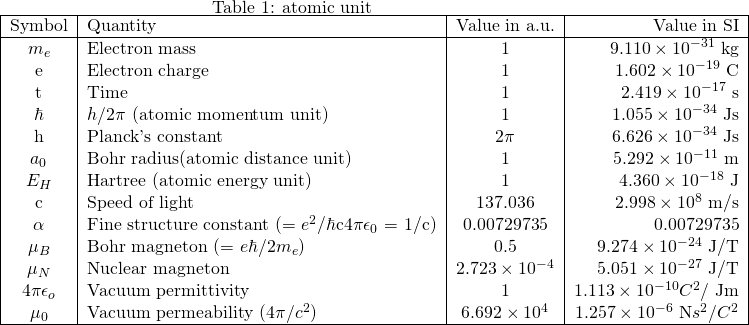#### Unit conversion

1 a.u. = 4.35981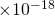J
1 a.u. = 27.21162 eV
1 a.u. = 627.510 kcal/mol
1 a.u. = 6.57981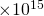Hz
1 a.u. = 45.5628 nm

#### Related Articles

This site uses Akismet to reduce spam. Learn how your comment data is processed.